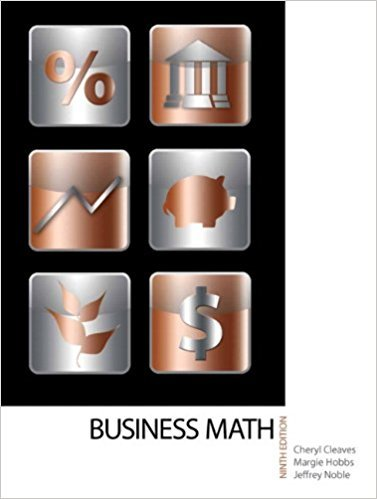×
×

# Solutions for Chapter 11-1: THE SIMPLE INTEREST FORMULA## Full solutions for Business Math, | 9th Edition

ISBN: 9780135108178Solutions for Chapter 11-1: THE SIMPLE INTEREST FORMULA

Solutions for Chapter 11-1
4 5 0 240 Reviews
10
2
##### ISBN: 9780135108178

Chapter 11-1: THE SIMPLE INTEREST FORMULA includes 16 full step-by-step solutions. This textbook survival guide was created for the textbook: Business Math, , edition: 9. This expansive textbook survival guide covers the following chapters and their solutions. Business Math, was written by and is associated to the ISBN: 9780135108178. Since 16 problems in chapter 11-1: THE SIMPLE INTEREST FORMULA have been answered, more than 19400 students have viewed full step-by-step solutions from this chapter.

Key Math Terms and definitions covered in this textbook
• Adjacency matrix of a graph.

Square matrix with aij = 1 when there is an edge from node i to node j; otherwise aij = O. A = AT when edges go both ways (undirected). Adjacency matrix of a graph. Square matrix with aij = 1 when there is an edge from node i to node j; otherwise aij = O. A = AT when edges go both ways (undirected).

• Augmented matrix [A b].

Ax = b is solvable when b is in the column space of A; then [A b] has the same rank as A. Elimination on [A b] keeps equations correct.

• Block matrix.

A matrix can be partitioned into matrix blocks, by cuts between rows and/or between columns. Block multiplication ofAB is allowed if the block shapes permit.

• Cholesky factorization

A = CTC = (L.J]))(L.J]))T for positive definite A.

• Column picture of Ax = b.

The vector b becomes a combination of the columns of A. The system is solvable only when b is in the column space C (A).

• Complex conjugate

z = a - ib for any complex number z = a + ib. Then zz = Iz12.

• Cross product u xv in R3:

Vector perpendicular to u and v, length Ilullllvlll sin el = area of parallelogram, u x v = "determinant" of [i j k; UI U2 U3; VI V2 V3].

• Distributive Law

A(B + C) = AB + AC. Add then multiply, or mUltiply then add.

• Dot product = Inner product x T y = XI Y 1 + ... + Xn Yn.

Complex dot product is x T Y . Perpendicular vectors have x T y = O. (AB)ij = (row i of A)T(column j of B).

• Fourier matrix F.

Entries Fjk = e21Cijk/n give orthogonal columns FT F = nI. Then y = Fe is the (inverse) Discrete Fourier Transform Y j = L cke21Cijk/n.

• Full column rank r = n.

Independent columns, N(A) = {O}, no free variables.

• Gram-Schmidt orthogonalization A = QR.

Independent columns in A, orthonormal columns in Q. Each column q j of Q is a combination of the first j columns of A (and conversely, so R is upper triangular). Convention: diag(R) > o.

• Hessenberg matrix H.

Triangular matrix with one extra nonzero adjacent diagonal.

• Hypercube matrix pl.

Row n + 1 counts corners, edges, faces, ... of a cube in Rn.

• Indefinite matrix.

A symmetric matrix with eigenvalues of both signs (+ and - ).

• Multiplication Ax

= Xl (column 1) + ... + xn(column n) = combination of columns.

• Orthogonal subspaces.

Every v in V is orthogonal to every w in W.

• Row picture of Ax = b.

Each equation gives a plane in Rn; the planes intersect at x.

• Singular matrix A.

A square matrix that has no inverse: det(A) = o.

• Spanning set.

Combinations of VI, ... ,Vm fill the space. The columns of A span C (A)!

×